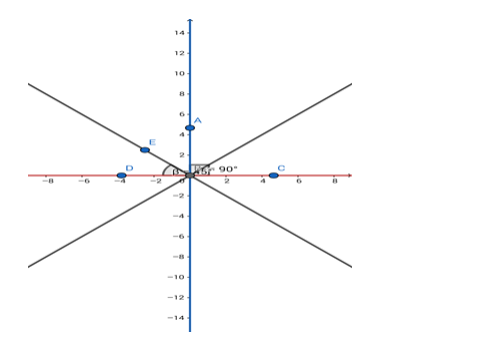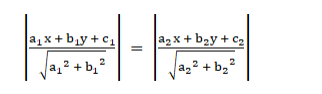# Find the equation of the bisectors of the angles between the coordinate axes.`
Question:

Find the equation of the bisectors of the angles between the coordinate axes.

Solution:Given: The straight lines are x = 0 and y = 0.

Formula to be used: If $\theta$ is the angle between two straight lines $a_{1} x+b_{1} y+c_{1}=0$ and $a_{2} x+b_{2} y+c_{2}=0$ then the equation of their angle bisector is$\therefore$ the equation of the angle bisectors is $\left|\frac{x}{\sqrt{1^{2}}}\right|=\left|\frac{y}{\sqrt{1^{2}}}\right|$

i.e. $x=\pm y$